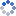加载中…漂流瓶jz
• 博客等级：• 博客积分：0
• 博客访问：217,810
• 关注人气：27
• 获赠金笔：0支
• 赠出金笔：0支
• 荣誉徽章：

## MATLAB中的 anova1()函数 单因素方差分析(2013-08-06 10:00:50)

### 字符串

Matlab 统计工具箱中单因素方差分析的命令是 anoval 。

（1 ）均衡数据

p=anoval(x)

x=[256     254     250     248    236
242     330     277     280    252
280     290     230     305    220
298     295     302     289    252];
p=anova1(x)

fcdf(2.262,4,15)=0.8891=1-p
Box 图反映了各组数据的特征。

（2）非均衡数据

p=anova1(x,group)
x 为向量，从第 1 组到第 r 组数据依次排列；group 为与 x 同长度的向量，标志 x 中数x=[1620    1580    1460    1500
1670    1600    1540    1550
1700    1640    1620    1610
1750    1720    1680    1800];
x=[x(1:4),x(16),x(5:8),x(9:11),x(12:15)];
g=[ones(1,5),2*ones(1,4),3*ones(1,3),4*ones(1,4)];
p=anova1(x,g)

<0.05，所以几种工艺制成的灯泡寿命有显著差异。

0